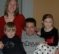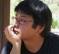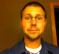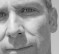## Best method for a large dot vectorStarted by 8 years ago13 replieslatest reply 8 years ago104 views

I am trying to compute a 274 sample dot vector in an FPGA (in #Verilog).  I have the clock cycles to compute it over many clocks, but I am having trouble meeting timing and figure I am doing something stupid in my methodology.

My overarching state machine is in a case statement and does have an catch-all state to if I get into some sort of weird state.  There is some setup and cleanup before/after the multiple, but basically what I am doing is:

    always @(posedge clock)
begin
en <= 1'b0;
case(state_counter)
..............

8'd4:
begin
if(counter < 8'd68)
begin
en <= 1'b1;
counter <= counter + 1'b1;
end
else
begin
state_counter <= 8'd5;
end
end

and then:

always @(en)
begin
if(en == 1'b1)
begin
if (sample_data_buffer_I[((counter)<<2)+sample_index+circ_buf_size-sync_pattern_size] !== 16'bx)

begin
corr_sum_I <= corr_sum_I + (sample_data_buffer_I[((counter)<<2)+sample_index+circ_buf_size-sync_pattern_size] * sync_pattern_I[((counter)<<2)]);

corr_sum_Q <= corr_sum_Q + (sample_data_buffer_Q[((counter)<<2)+sample_index+circ_buf_size-sync_pattern_size] * sync_pattern_Q[((counter)<<2)]);

end

if (sample_data_buffer_I[(((counter)<<2)+2'd1)+sample_index+circ_buf_size-sync_pattern_size] !== 16'bx)

begin

corr_sum_I <= corr_sum_I + (sample_data_buffer_I[(((counter)<<2)+2'd1)+sample_index+circ_buf_size-sync_pattern_size] * sync_pattern_I[(((counter)<<2)+2'd1)]);

corr_sum_Q <= corr_sum_Q + (sample_data_buffer_Q[(((counter)<<2)+2'd1)+sample_index+circ_buf_size-sync_pattern_size] * sync_pattern_Q[(((counter)<<2)+2'd1)]);

end

if (sample_data_buffer_I[(((counter)<<2)+2'd2)+sample_index+circ_buf_size-sync_pattern_size] !== 16'bx)

begin

corr_sum_I <= corr_sum_I + (sample_data_buffer_I[(((counter)<<2)+2'd2)+sample_index+circ_buf_size-sync_pattern_size] * sync_pattern_I[(((counter)<<2)+2'd2)]);

corr_sum_Q <= corr_sum_Q + (sample_data_buffer_Q[(((counter)<<2)+2'd2)+sample_index+circ_buf_size-sync_pattern_size] * sync_pattern_Q[(((counter)<<2)+2'd2)]);

end

if (sample_data_buffer_I[(((counter)<<2)+2'd3)+sample_index+circ_buf_size-sync_pattern_size] !== 16'bx)

begin

corr_sum_I <= corr_sum_I + (sample_data_buffer_I[(((counter)<<2)+2'd3)+sample_index+circ_buf_size-sync_pattern_size] * sync_pattern_I[(((counter)<<2)+2'd3)]);

corr_sum_Q <= corr_sum_Q + (sample_data_buffer_Q[(((counter)<<2)+2'd3)+sample_index+circ_buf_size-sync_pattern_size] * sync_pattern_Q[(((counter)<<2)+2'd3)]);

end

end

end



Can anyone tell me a more elegant way of doing this (also, The next state does the final 2 muliplications and the state after that sums them all up).

[ - ]If the FPGA family you are using does not have dedicated math resources (add, mul, mac) you would be trying to synthesize everything out of basic LUTs. It will be extremely difficult to close timing unless you heavily pipeline your implementation. You should be able to get a handle on your timing problems by looking at your critical paths, your timing analysis process should be able to give you this information.

I notice that you have a conditional statement that looks for some value to not be in an unknown state. I don't know what you expect logic synthesis to do with this, there are no X states in real hardware. If correctness of simulation depends on this construct, your synthesized gate-level logic may be broken. Typically you would want to either reset registered state as necessary, or flush unknowns out of your data path by applying known input for some number of clocks. There is the obvious feedback case where next state depends on current state, you either have to reset related state or inject a known value into the feedback path which is really a type of reset.

A little bit of methodology goes a long way.

Organize your code into modules, each module contains a) port and variable declarations, b) combinational logic definition, and c) register assignments. Name clocked register state variables (flops) in a specific way, eg. r_foo, r_bar, etc. These variables are always Verilog register types. Similarly name combinational variables (wires) as c_abc, c_xyz, etc. These variables can be Verilog wire or register types.

The combinational logic section is where you define the cones of logic that produce a value for each c_ variable from other c_ and r_ variables, these should all be continuous or procedural assignments using =. This is where all the work is done.

The register assignment section is trivial and should consist only of non-blocking procedural assignments that operate on posedge clock (and possibly on reset). Here you simply assign the output of a cone of logic to a register, for example:

r_foo <= c_foo;

This is the only place you should use <= assignments. This code structure reflects the structure of a synchronous digital system and does not depend on micro-time behavior. Code written this way will be highly portable and will behave as expected.

If you have a heavily pipelined implementation it is useful to name variables according to their pipeline level, for example r1_operand, r2_partialsum, r3_product and so on.

You can get away without such coding discipline in small relatively simple designs. However, as a design grows in size and complexity this methodology simplifies the design and debug process. Code, timing reports and simulation traces are a lot easier to read and understand. I was introduced to this methodology several decades ago by some very capable senior designers at a company that successfully designed and produced computers. It will serve you well.

[ - ]I agree with mathewbarr.

I'm also having trouble getting the code formatted into a form in which I can digest it. It also doesn't help that I'm far more proficient in VHDL but my initial reaction is that too many calculations are being done in single clock cycles. Also, the structure of the code does not make it easy to break the operations apart as necessary as timing issues are identified.

I find that hardware coding in very simple, almost stupid sequences of operations really helps in producing a working system and one that can be optimized when your fmax falls short of the requirement.

Perhaps the code could be linked to a public dropbox or stashed in github?

[ - ]Thank you for all the info @matthewbarr.  I am always looking to improve the way I do things, so I appreciate the feedback.  Is there some code online somewhere that utilizes your methodology?  I've read through your write-up twice, but wouldn't mind seeing it in action to make sure I am on the same page.

[ - ]For different multiplier architecture mechanism to improve speed, you may refer to the first chapter of Steve Kilts book "Advanced FPGA Design". It gives good examples.

[ - ]The OP didn't specify the bits widths in the design.  In general, you will be best off using the hard multipliers in an FPGA.  I would be surprised if this design was not targeted for a device with four or more math blocks (I believe both Altera and Xilinx call them a DSP something or another).

Now, if the full design has used up all the resources that is another issue and exploring alternate multiplier/mac structures might help.

[ - ]I will ping a few members in case they can help you: @barcelonajack, @zynqer, @asser, @melpin94, @elliotxu, @maiatec, @matthewbarr, @M65C02A, @thorndbear, @gcary, @allenkrell, @picoskop, @divner, @cfelton

[ - ]Thanks so much.  Also, is there a way to wrap the code so that the forum knows that it was code?  I had a heck of a time formating it, and it still looks rather gross!).

[ - ]@garengllc instead of dumping your complete code in the post, it is probably best to isolate a snippet of the code (digestable in the post) and then link to the full code source.

A couple additional pieces of information would be useful: 1) what is the clock rate that you are trying to operate the circuit; 2) what is the sample rate you are trying to operate at?

[ - ]@garengllc,  I should clarify, you can break you code down like this:

The following is my state-machine and it does XYZ

always @(posedge clock) begin
// state-machine logic
end


The state-machine controls the data-path, which does XYZ

always @(posedge clock) begin
// data-path
end

[ - ]I think I am missing something here, let me make sure I got it.  Does something like this make sense:

always>@(posedge clock)begin
case(sel)
begin
1'd1: sel <= 1'd2
1'd2: sel <= 1'd3
1'd3: sel <= 1'd1
end
end

always @(posedge clock)
begin
if(sel == 1'd1)
//do something
else if (sell == 1'd2)
//do something else
else
//last thing
end        
[ - ]Good points @cfelton.  It is a snippet I put in there, but a link to the full code would probably have been smart.

My clock rate is 184.32MHz and my sample rate is 1.92MSPS.  SO in this case, I have 96 clocks to get my work done and I am utilizing about 80 or so.

[ - ]